# Count Number of Times a Word Appears in a Cell – Excel & Google Sheets

This tutorial demonstrates how to count the number of times a word appears in a cell.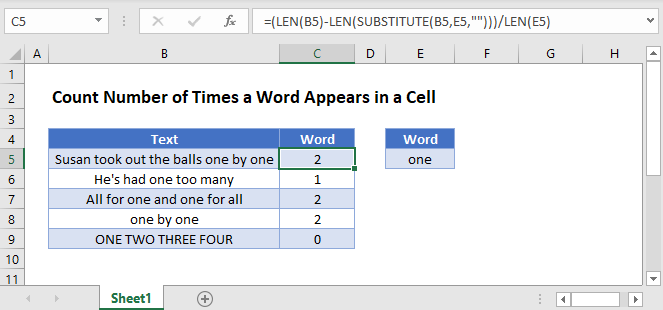## Count Specific Words

To count the number of times a word appears in a cell, we will use the LEN and SUBSTITUTE Functions.

``=(LEN(B3)-LEN(SUBSTITUTE(B3,E3,"")))/LEN(E3)``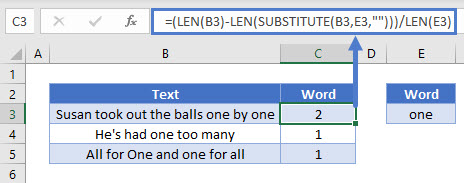In the example above, our objective is to count the number of times the word “one” appears in each cell.

The formula calculates the length of the original cell and then gets the length of the cell after substituting the word to be counted with a blank, subtracting the two lengths. The result is then divided by the length of the word, this gives us the number of times a word appears in a cell.

Let’s walk through the formula by breaking it down to its individual functions.

## The LEN Function

First, we will use the LEN Function to count the total number of characters in the text string

``=LEN(B3)``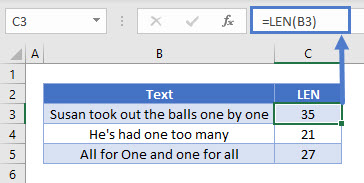The formula above returned the count of the characters in the text string including the spaces.

## The SUBSTITUTE Function

Next, we will use the SUBSTITUTE Function to remove the word “one” from the text string.

``=SUBSTITUTE(B3,F3,"")``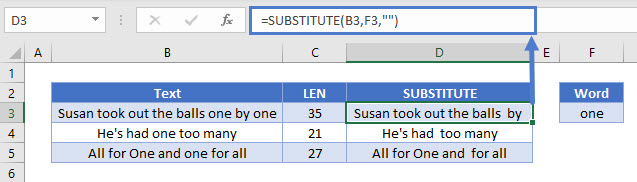The SUBSTITUTE Function is case sensitive, so the word needs it to be in the same case (upper or lower case) as it is in the cell for it to be substituted.

Using the formula above, every instance of the word “one” in the text strings was replaced with a blank.

## The Length of Substituted Text

Now we can use the LEN Function to count the number of characters left, after removing all instances of the word (“one”) from the text.

``=LEN(D3)``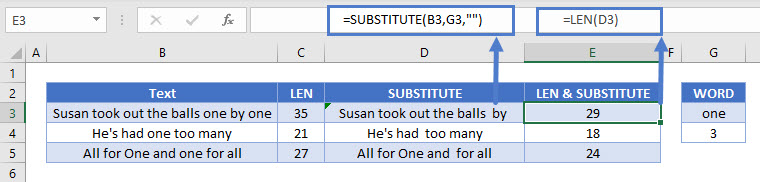## The Length of Characters Removed

To determine the number of characters that were removed from the original text, we can take the difference between the two LEN Function results.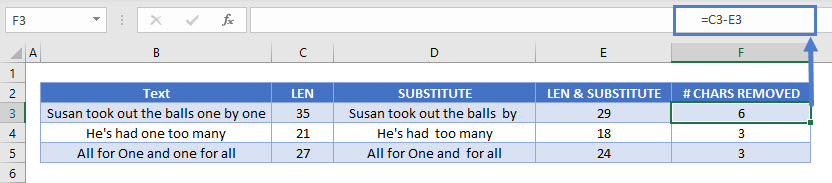Dividing this by the character length of the word (“one” has 3 characters), gives us the number of times the word appears in each cell.

``=LEN(G3)``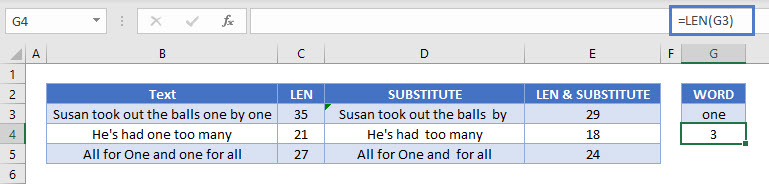Now that we’ve gone through the individual parts of the formula, bringing it all together gives us our initial formula. It returns the number of times the word “one” appears in each cell.

``=(LEN(B3)-LEN(SUBSTITUTE(B3,E3,"")))/LEN(E3)``## Count Times Word Appears In Cell in Google Sheets

These formulas work exactly the same in Google Sheets as in Excel.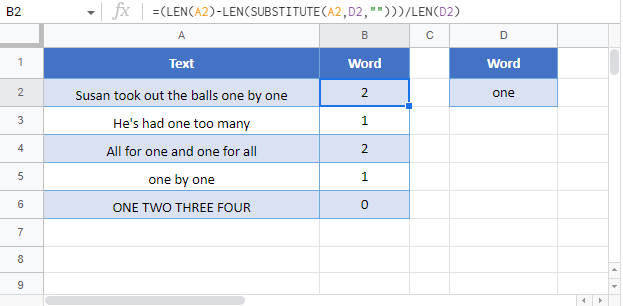### Excel Practice Worksheet

Practice Excel functions and formulas with our 100% free practice worksheets!